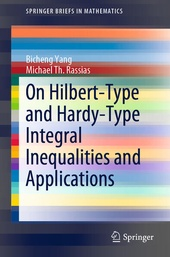# On Hilbert-Type and Hardy-Type Integral Inequalities and Applications

Springer (Verlag)
• 1. Auflage
• |
• erschienen am 30. September 2019

• Buch
• |
• Softcover
• |
• 156 Seiten
978-3-030-29267-6 (ISBN)

This book is aimed toward graduate students and researchers in mathematics, physics and engineering interested in the latest developments in analytic inequalities, Hilbert-Type and Hardy-Type integral inequalities, and their applications. Theories, methods, and techniques of real analysis and functional analysis are applied to equivalent formulations of Hilbert-type inequalities, Hardy-type integral inequalities as well as their parameterized reverses. Special cases of these integral inequalities across an entire plane are considered and explained. Operator expressions with the norm and some particular analytic inequalities are detailed through several lemmas and theorems to provide an extensive account of inequalities and operators.
 Reihe: Auflage: 1st ed. 2019 Sprache: Englisch Verlagsort: Cham | Schweiz Verlagsgruppe: Springer International Publishing Zielgruppe: Für Beruf und Forschung Illustrationen: 1 s/w Abbildung | 1 Illustrations, black and white; X, 145 p. 1 illus. Maße: Höhe: 235 mm | Breite: 155 mm | Dicke: 8 mm Gewicht: 248 gr Schlagworte: ISBN-13: 978-3-030-29267-6 (9783030292676) DOI: 10.1007/978-3-030-29268-3weitere Ausgaben werden ermittelt
1. Introduction.- 2.Equivalent Statements of Hilbert-type integral inequalities.- 3.Equivalent Statements of the Reverse Hilbert-Type Integral Inequalities.- 4. Equivalent Statements of Hardy-Type Integral Inequalities.- 5.Equivalent Property of the Reverse Hardy-Type Integral Inequalities.- References.
"This monograph could be useful for graduate students of mathematics, physics and engineering sciences, or to anyone interested in this active field of research." (J. Sandor, Mathematical Reviews, May, 2020)
This book is aimed toward graduate students and researchers in mathematics, physics and engineering interested in the latest developments in analytic inequalities, Hilbert-Type and Hardy-Type integral inequalities, and their applications. Theories, methods, and techniques of real analysis and functional analysis are applied to equivalent formulations of Hilbert-type inequalities, Hardy-type integral inequalities as well as their parameterized reverses. Special cases of these integral inequalities across an entire plane are considered and explained. Operator expressions with the norm and some particular analytic inequalities are detailed through several lemmas and theorems to provide an extensive account of inequalities and operators.
Schweitzer Klassifikation
• Mathematik
•Analysis
•Funktionalanalysis
• Mathematik
•Analysis
•Reelle Analysis
• Mathematik
•Angewandte Mathematik
•Numerische Mathematik
Thema Klassifikation
Newbooks Subjects & Qualifier
• Mathematik | Informatik
•Mathematik
•Geometrie
•Dynamische Systeme
• Mathematik | Informatik
•Mathematik
•Mathematische Analysis
•Funktionalanalysis
• Mathematik | Informatik
•Mathematik
•Mathematische Analysis
•Reelle Analysis
DNB DDC Sachgruppen
Dewey Decimal Classfication (DDC)
• Science
•Mathematics
•Analysis
• Science
•Mathematics
•Mathematics
• Science
•Mathematics
•Numerical analysis
BIC Classifikation
• Mathematics & science
•Mathematics
•Applied mathematics
•Mathematical modelling
• Mathematics & science
•Mathematics
•Applied mathematics
•Nonlinear science
• Mathematics & science
•Mathematics
•Calculus & mathematical analysis
•Numerical analysis
• Mathematics & science
•Mathematics
•Geometry
•Fractal geometry
BISAC Classifikation
Warengruppensystematik 2.0

Sofort lieferbar

 53,49 € inkl. 5% MwSt.